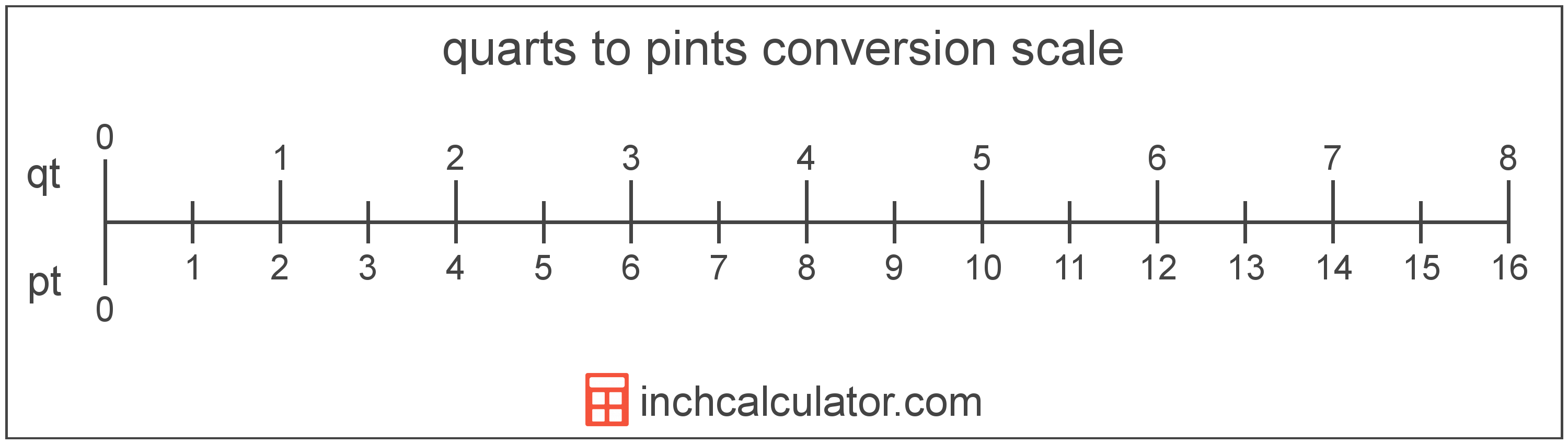# Quarts to Pints Conversion

Enter the volume in quarts below to get the value converted to pints.

Results in Pints:1 qt = 2 pt
Do you want to convert pints to quarts?

## How to Convert Quarts to PintsTo convert a quart measurement to a pint measurement, multiply the volume by the conversion ratio.

Since one quart is equal to 2 pints, you can use this simple formula to convert:

pints = quarts × 2

The volume in pints is equal to the quarts multiplied by 2.

For example, here's how to convert 5 quarts to pints using the formula above.
5 qt = (5 × 2) = 10 pt

### How Many Pints are in a Quart?

There are 2 pints in a quart, which is why we use this value in the formula above.

1 qt = 2 pt

## Quarts

The US liquid quart is a unit of fluid volume equal to one fourth of a gallon, two pints, or four cups. The liquid quart should not be confused with the dry quart (US) or the imperial quart, which are different units.

The quart is a US customary unit of volume. Quarts can be abbreviated as qt; for example, 1 quart can be written as 1 qt.

## Pints

The US liquid pint is a unit of fluid volume equal to one-eighth of a gallon, one-half of a quart, or two cups. The liquid pint should not be confused with the dry pint (US) or the imperial pint, which are different units.

The pint is a US customary unit of volume. Pints can be abbreviated as pt; for example, 1 pint can be written as 1 pt.

## Quart to Pint Conversion Table

Quart measurements converted to pints
Quarts Pints
1 qt 2 pt
2 qt 4 pt
3 qt 6 pt
4 qt 8 pt
5 qt 10 pt
6 qt 12 pt
7 qt 14 pt
8 qt 16 pt
9 qt 18 pt
10 qt 20 pt
11 qt 22 pt
12 qt 24 pt
13 qt 26 pt
14 qt 28 pt
15 qt 30 pt
16 qt 32 pt
17 qt 34 pt
18 qt 36 pt
19 qt 38 pt
20 qt 40 pt
21 qt 42 pt
22 qt 44 pt
23 qt 46 pt
24 qt 48 pt
25 qt 50 pt
26 qt 52 pt
27 qt 54 pt
28 qt 56 pt
29 qt 58 pt
30 qt 60 pt
31 qt 62 pt
32 qt 64 pt
33 qt 66 pt
34 qt 68 pt
35 qt 70 pt
36 qt 72 pt
37 qt 74 pt
38 qt 76 pt
39 qt 78 pt
40 qt 80 pt